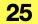Describes the Structural Analytical Model Analytical Edge Load settings.

```    typedef enum {
API_Force,
API_Moment
```
```    typedef struct {
API_AnalyticalCoordSysDirection         coordinateSystemType;
API_Vector3D                            startValue;
API_Vector3D                            endValue;
```

#### Members

loadCaseIdThe guid of the connected Load Case.
loadForceTypeThe force type of the Analytical Edge Load. The edge force can be defined in three main directions (global or local coordinate system). An edge of the structure may be subject to a moment load. The load is defined by the direction and size of the moment.
loadDistributionTypeThe load may be either constant along the 1D member or linearly variable (trapezoidal).
coordinateSystemTypeDefines the co-ordinate system of the member in which the load is applied. For ” Local”, coordinate system is defined by the member where is load applied
startValueThe start force/moment value of the Analytical Edge Load in the X, Y and Z directions.
endValueThe end force/moment value of the Analytical Edge Load in the X, Y and Z directions.

#### Requirements

Version: API 25 or later